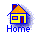# Pol4Pal CalculatorEnter a numerical palindrome (sequence of positive natural numbers separated by spaces)

Receive the polynomial that gives all possible n ∈ N such that the continued fraction of n starts with [z, «palindrome», 2z] for some z

 First part (first half or elements before the middle) Middle element (leave blank if the length is even) Your palindrome is

## How to use this calculator

1. Choose a palindrome (ex.: "3 4 1 4 3" or "2 13 13 2")
2. If it has even length
1. Enter the first half (2 13 13 2)
2. Leave "Middle element" blank
3. If it has odd length
1. Enter the elements before the middle (3 4 1 4 3)
2. Enter the middle element (3 4 1 4 3)
4. Click Calculate to get the polynomial

## How polynomials are calculated

Palindrome to polynomial mapping:

 Palindrome Polynomial [   ] (Empty) f(x) = x2 + 1 x ∈ N [2m] f(x) = (m2x + 1)x x > 0 [2m − 1] f(x) = ((2m − 1)2x + 2) x > 0 [1, 2m − 1, 1] f(x) = ((2m + 1)2x + (2m + 1)2 − 2)(x + 1) x ∈ N

For the other cases, read the paper

## Credits

Mathematics
Andrzej Solecki

Informatics
Igor da Silva Solecki